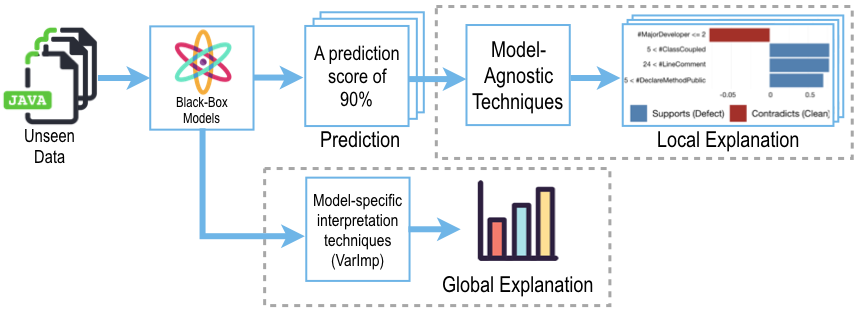# Techniques for Generating Explanations¶

Prior studies often leverage white-box AI/ML techniques, such as decision trees and decision rules. The transparency of such white-box AI/ML techniques allows us to meaningfully understand the magnitude of the contribution of each metric on the learned outcomes by directly inspecting the model components. For example, the coefficients of each metric in a regression model, paths in a decision tree, or rules of a decision rule model

In contrast, white-box AI/ML techniques are often less accurate than complex black-box AI/ML techniques and often generate generic explanations (e.g., one decision node may cover 100 instances). Recently, model-agnostic techniques (e.g., LIME [RSG16b] and BreakDown [GB19]) have been used to explain the predictions of any black-box AI/ML models at an instance level. Below, we provide the definition of (1) model-specific techniques for generating global explanations and (2) model-agnostic techniques for generating local explanations.Fig. 1 An illustration of model-specific techniques for generating global explanations and model-agnostic techniques for generating local explanations.

Model-specific explanation techniques focus on explaining the entire decision-making process of a specific black-box model. For example, an ANOVA analysis for logistic regression and a variable importance analysis for random forests. However, such global explanations are often derived from black-box models that are constructed from training data, which are not specific enough to explain an individual prediction.

Model-agnostic techniques (i.e., local explanation techniques), on the other hand, focus on explaining an individual prediction by diagnosing a black-box model. Unlike model-specific explanation techniques described above, the great advantage of model-agnostic techniques is their flexibility. Such model-agnostic techniques can (1) interpret any classification techniques (e.g., regression, random forest, and neural networks); (2) are not limited to a certain form of explanations (e.g., feature importance or rules); and (3) are able to process any input data (e.g., features, words, and images [RSG16a]).